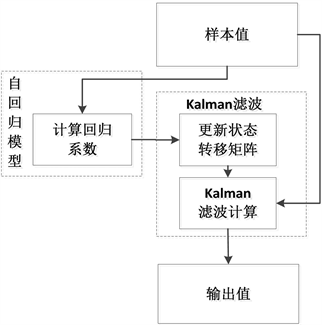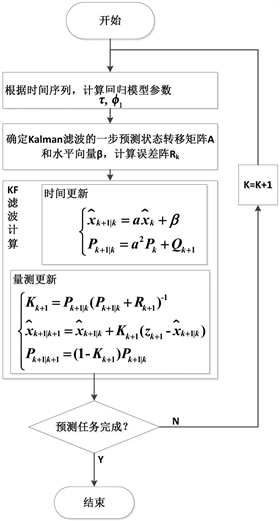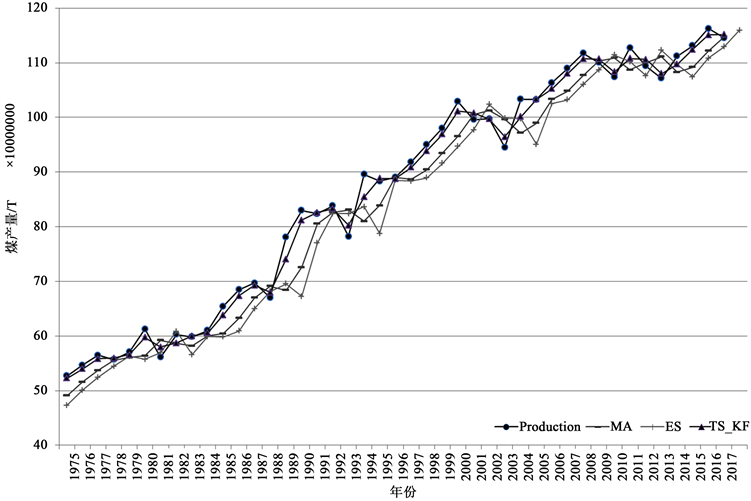﻿ 一种短时序Kalman滤波决策优化预测新方法

# 一种短时序Kalman滤波决策优化预测新方法A New Decision Optimization Prediction Method Based on Short-Term Time Series and Kalman Filter

Abstract: In order to make scientific and agile decision, a new short-term prediction TS_KF (Time Se-ries-Kalman Filter) method is proposed based on the combination of time series analysis and Kalman Filter. Aiming to improve the prediction precision and decrease the calculation complexity, a prediction model is built using Kalman filter to describe the prediction process, and the auto regression for time series analysis is utilized to renew and optimize the state transfer matrix, which is the key parameter for Kalman Filter. A coal production prediction test is conducted by comparison with some typical time series prediction method, and the results show that the TS_KF prediction method in this paper has significantly enhanced the prediction precision while keeping the same low calculation complexity. The result gives a strong proof for the effectiveness of the new TS_KF prediction method.

1. 引言

2. TS_KF预测方法

2.1. 预测模型框架

TS_KF预测方法以Kalman滤波预测模型为基础，预测模型能保持Kalman滤波本身的迭代收敛特性，在使用过程中通过样本的积累实现逐步收敛。预测模型框架如图1Figure 1. Predictive model frameworkTable 1. Comparison of different prediction methods

• 不必进行前期大量样本值的训练，而将此过程融入到运行过程中，减少了模型应用过程中的前期准备工作量；

• 在使用过程中可以对样本值进行滤波，减小样本本身的误差，这是其它模型没有实现的。

2.2. 预测模型的构建

$\left\{\begin{array}{l}{x}_{{}_{k}}=A{x}_{k-1}+B{u}_{k}+{w}_{k-1}\\ {z}_{k}=H{x}_{k}+{v}_{k}\end{array}$ (1)

$\left\{\begin{array}{l}{w}_{k-1}~N\left(0,Q\right)\\ {v}_{k}~N\left(0,R\right)\end{array}$ (2)

$\left\{\begin{array}{l}{x}_{k}=a{x}_{k-1}+{w}_{k-1}\\ {z}_{k}={x}_{k}+{v}_{k}\end{array}$ (3)

${\stackrel{˜}{y}}_{t}={\varphi }_{1}{\stackrel{˜}{y}}_{t-1}+{\varphi }_{2}{\stackrel{˜}{y}}_{t-2}+\cdot \cdot \cdot +{\varphi }_{p}{\stackrel{˜}{y}}_{t-p}+\xi$ (4)

${\stackrel{˜}{y}}_{k}={\varphi }_{1}{\stackrel{˜}{y}}_{k-1}+\xi$ (5)

$\mu$${y}_{k}$ 的期望水平的情况下，将 ${\stackrel{˜}{y}}_{k}={y}_{k}-\mu$ 代入到(5)，可得AR(1)的以下等效过程：

${y}_{k}={\varphi }_{1}{y}_{k-1}+\tau +\xi$ (6)

$\left\{\begin{array}{l}\stackrel{¯}{y}=\frac{1}{S}{{\sum }_{i=0}^{S}y}_{k-i}\\ {\varphi }_{1}=\frac{{{\sum }_{i=0}^{S-1}{y}_{k-i}y}_{k-i-1}-\left(S-1\right){\stackrel{¯}{y}}^{2}}{{\sum }_{i=0}^{S-1}{y}_{k-i-1}^{2}-\left(S-1\right){\stackrel{¯}{y}}^{2}}\\ \tau =\left(1-{\varphi }_{1}\right)\stackrel{¯}{y}\end{array}$ (7)

$\left\{\begin{array}{l}{x}_{{}_{k}}=a{x}_{k-1}+\beta +{w}_{k-1}\\ {z}_{k}={x}_{k}+{v}_{k}\end{array}$ (8)

$\left\{\begin{array}{l}{\sigma }^{2}={{\sum }_{i=0}^{S}\left[{\stackrel{^}{y}}_{i}-\left({\varphi }_{1}{\stackrel{^}{y}}_{i-1}+\tau \right)\right]}^{2}\\ {R}_{k}=diag\left({\sigma }^{2}\right)={\sigma }^{2}\end{array}$ (9)

2.3. 算法描述Figure 2. Predictive algorithm flow

3. 实验研究Table 2. Comparison experimental parameter setting of different prediction methodsTable 3. A detailed list of predicted statistical dataTable 4. Error statistics of different prediction modelsFigure 3. Comparison of forecast effect of coal yield using various predictive methods

4. 结论

1) 综述了决策中现有的典型预测方法，阐述了不同预测方法的应用场合和相互关系。统计时间序列方法主要用于短期预测，是决策敏捷性的重要支撑，因此着重对短期时间序列预测进行了研究。

2) 为提升决策的敏捷性和科学性，以短时段数据的时间序列为研究对象，提出了将时间序列模型和Kalman滤波模型相结合的TS_KF预测方法，并从预测准确性和计算复杂度上优化预测方法。TS_KF方法具有模型简洁、有效、计算复杂度低等特点。

3) 以煤产量数据作为时间序列样本，采用与典型的时间序列预测方法(MA和ES)对比的方法，设计了实验验证算例，以验证TS_KF方法的有效性。结果表明，在保持计算复杂度同水平的前提下，TS_KF方法能极大的提高预测的准确性。

NOTES

*通讯作者。

 杨庆芳, 赵小辉, 郑黎黎. 基于模型预测控制的环形交叉口信号配时方法[J]. 浙江大学学报(工学版), 2018, 52(1): 117-124.

 Pinto, R. and Gaiardelli, P. (2013) Setting Forecasting Model Parameters Using Unconstrained Direct Search Methods: An Empirical Evaluation. Expert Systems With Applications, 40, 5331-5340.
https://doi.org/10.1016/j.eswa.2013.03.044

 埃文斯. 数据、模型与决策[M]. 北京: 中国人民大学出版社, 2012.

 Dunn, D.W., Buelow, J.M., Austin, J.K., et al. (2004) Development of Syndrome Severity Scores for Pediatric Epilepsy. Epilepsia, 45, 661-666.
https://doi.org/10.1111/j.0013-9580.2004.53903.x

 Whitehead, D. (2008) An International Delphi Study Examining Health Promotion and Health Education in Nursing Practice, Education and Policy. Journal of Clinical Nursing, 17, 891-900.
https://doi.org/10.1111/j.1365-2702.2007.02079.x

 Weiss, M., Junginger, M., Patel, M.K., et al. (2010) A Review of Expe-rience Curve Analyses for Energy Demand Technologies. Technological Forecasting and Social Change, 77, 411-428.
https://doi.org/10.1016/j.techfore.2009.10.009

 Hsu, L. and Wang, C. (2007) Forecasting the Output of Integrated Circuit Industry Using a Grey Model Improved by the Bayesian Analysis. Technological Forecasting and Social Change, 74, 843-853.
https://doi.org/10.1016/j.techfore.2006.02.005

 Yuan, M. and Lin, Y. (2006) Model Selection and Estimation in Regression with Grouped Variables. Journal of The Royal Statistical Society Series B-Statistical Methodology, 68, 49-67.
https://doi.org/10.1111/j.1467-9868.2005.00532.x

 Yu, H.L. and Macgregor, J.F. (2003) Multivariate Image Analysis and Regression for Prediction of Coating Content and Distribution in the Production of Snack Foods. Chemometrics and Intelligent La-boratory Systems, 67, 125-144.
https://doi.org/10.1016/S0169-7439(03)00065-0

 Atiya, A.F. (2001) Bankruptcy Prediction for Credit Risk Using Neural Networks: A Survey and New Results. IEEE Transactions on Neural Networks, 12, 929-935.
https://doi.org/10.1109/72.935101

 Choi, T.M., Yu, Y. and Au, K.F. (2011) A Hybrid SARIMA Wavelet Transform Method for Sales Forecasting. Decision Support Systems, 51, 130-140.
https://doi.org/10.1016/j.dss.2010.12.002

 Min, S.H., Lee, J. and Han, I. (2006) Hybrid Genetic Algorithms and Support Vector Machines for Bankruptcy Prediction. Expert Systems with Ap-plications, 31, 652-660.
https://doi.org/10.1016/j.eswa.2005.09.070

 Sheikhan, M. and Mohammadi, N. (2012) Neural-Based Electricity Load Forecasting Using Hybrid of GA and ACO for Feature Selection. Neural Computing & Applications, 21, 1961-1970.
https://doi.org/10.1007/s00521-011-0599-1

 Hadavandi, E., Shavandi, H., Ghanbari, A., et al. (2012) Developing a Hybrid Artificial Intelligence Model for Outpatient Visits Forecasting in Hospitals. Applied Soft Computing, 12, 700-711.
https://doi.org/10.1016/j.asoc.2011.09.018

 Carpinone, A., Giorgio, M., Langella, R. and Testa, A. (2015) Markov Chain Modeling for Very-Short-Term Wind Power Forecasting. Electric Power Systems Research, 122, 152-158.
https://doi.org/10.1016/j.epsr.2014.12.025

 Chudik, A., Grossman, V. and Hashem Pesaran, M. (2016) A Multi-Country Approach to Forecasting Output Growth Using PMIs. Journal of Econometrics, 192, 349-365.
https://doi.org/10.1016/j.jeconom.2016.02.003

 宗群, 赵占山, 商安娜. 基于季节自回归单整移动平均模型的电梯交通流递归预测方法[J]. 天津大学学报, 2008, 41(8): 653-659.

 吴德会. 动态指数平滑预测方法及其应用[J]. 系统管理学报, 2008, 17(2): 151-155.

Top